# 第04次作业-树

## 1.学习总结

### 1.1树结构思维导图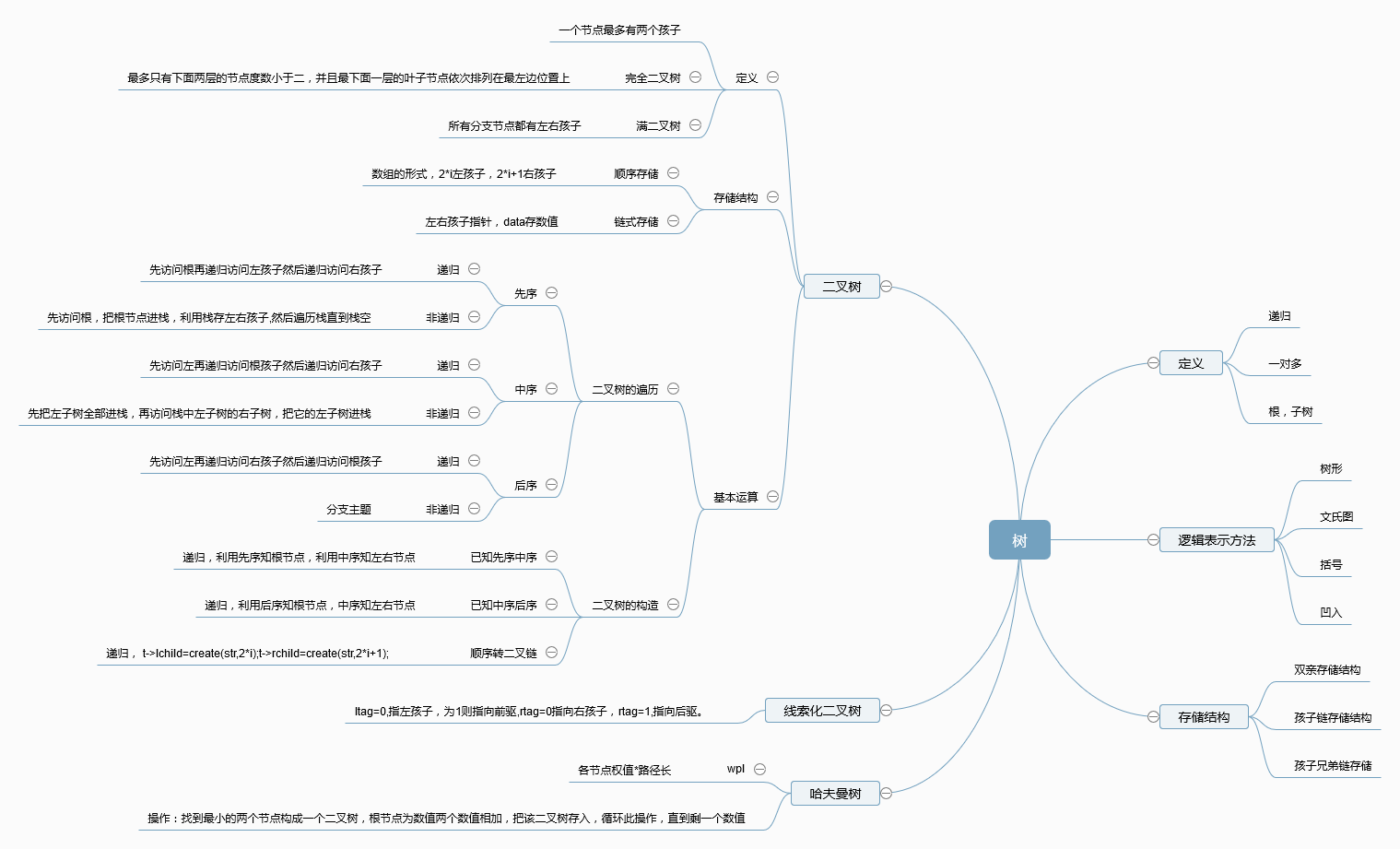### 1.2 树结构学习体会

树的结构是一种非线性结构，一对多，定义也是递归的，有一个根结点和任意个子树。



## 2.PTA实验作业

### 2.1题目一 6-4 jmu-ds-表达式树（25 分）

2.2 设计思路（伪代码或流程图）

    void InitExpTree(BTree &T,string str)
{
初始化两个栈s1,s2，分别存操作数和运算符；
int i=0;BTree s;
while (i<str.size())
do
if(str[i]是操作数）
then
新建节点s,存入操作数，并置左右孩子为空；
s进栈s1；

else
then
if(s2为空）
then
新建节点s,存入运算符，并置左右孩子为空；
进栈s2；
else
if(str[i]的优先级大于s2.top())
then
新建节点s,存入运算符，并置左右孩子为空；
进栈s2；
else
then
if(str[i]是'）'）
then
while (s2.top()!='(')
s2.top()->rchild=s1.top();
s1.pop()
s2.top()->lchild=s1.top();
s1.pop()
s2.pop()
end while
else
then
while(s2.top()!='('并且s2.top()优先级小于str[i])
重复上面遇到'('的操作，为s2配左右孩子
end while
end while
}
double EvaluateExTree(BTree T)
{
if(T->data为数字字符）
return T->data-'0';
if(T为空）return 0；
else
{
switch(T->data)
{
case '+':return 左子树的值+右子树的值；break;
case '-':return 左子树的值-右子树的值；break;
case '*':return 左子树的值*右子树的值；break;
case '/':if(右子树空不为0）
return 左子树的值/右子树的值；break;
else 打印除零错误语句
}


### 2.3 代码截图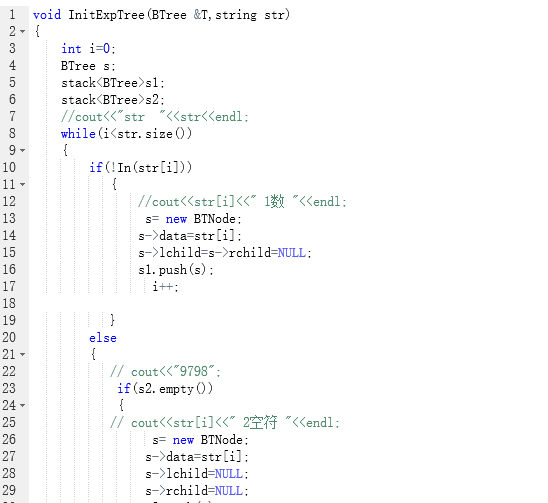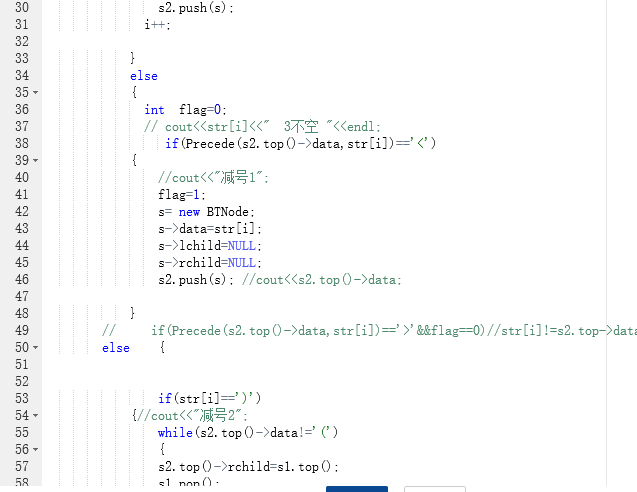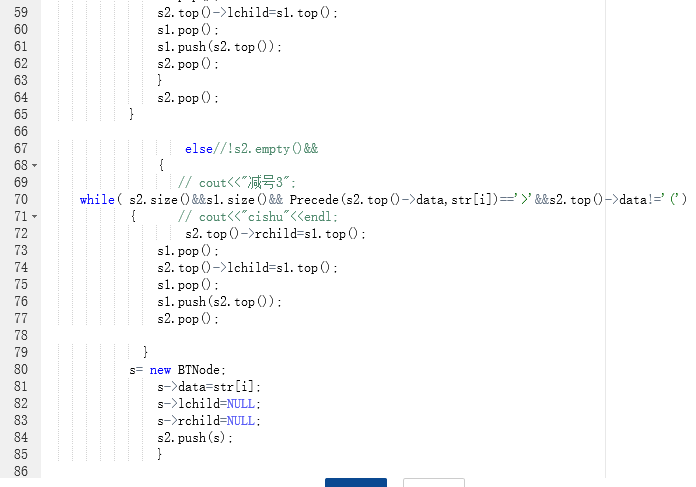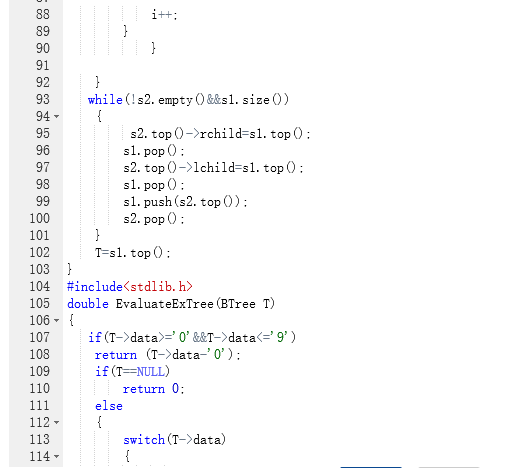### 2.4 PTA提交列表说明。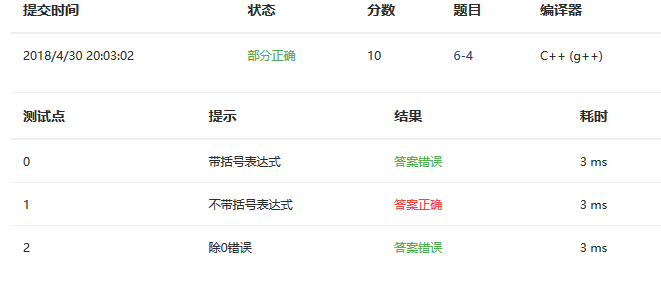• 错误原因
例如输入1/0
会出现如下情况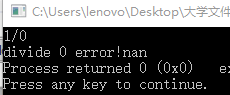• 解决方法：询问同学后发现，把return 0转换成exit(0)，并添加一个stdlib.h,就不会出现了，exit功能是关闭所有文件，终止正在执行的进程。

• 错误原因
在优先级小于栈顶且不是右括号的时候，出栈应该出到栈顶元素优先级小于str[i]，或遇到左括号，才能停止。不能只出一次
• 解决方法
增加while语句
 while( s2.size()&&s1.size()&& Precede(s2.top()->data,str[i])=='>'&&s2.top()->data!='(')


### 2.1题目二 7-7 修理牧场（25 分）

2.2 设计思路（伪代码或流程图）

 queue<int>q;
cin>>n;
int min1,min2;
for i=0 to n-1
cin>>p,p全部进队q
while (q.size()-1)
do
min1=q.front();q.pop()
min2=q.front();q.pop()

for i=0 to n-1
if(队首元素<min1)
then
q.push(min2)
min2的值等于min1
min1的值为队首元素
q.pop();

elseif(队首元素<min2)
then
q.push(min2)
min2=队首元素
q.pop()

else
q.push(队首）；
q.pop();
endfor

sum=min1+min2
q.push(sum)
end while



### 2.3 代码截图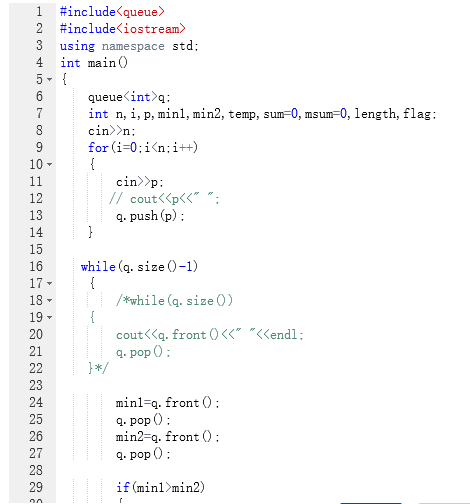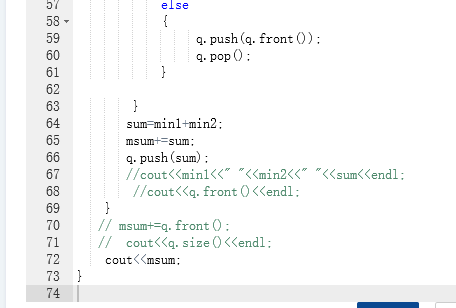### 2.4 PTA提交列表说明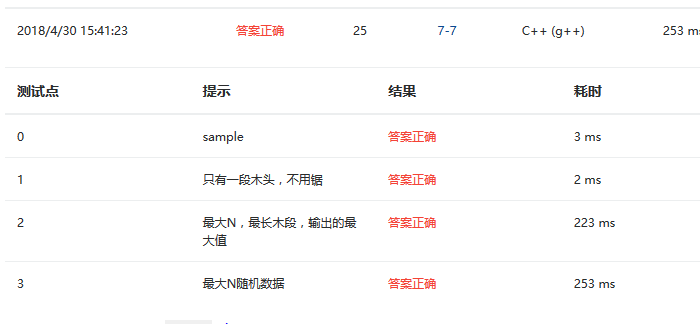### 2.2 设计思路（伪代码或流程图）

int sum  全局变量
btree create(string str ,int i )//顺序转化为二叉链
{
btree t;
t=new tnode;
if(i为负数||i>=str[i]) return NULL//注意>=
t->data=str[i];
t->rchild=(str,2*i+1)//下标关系
t->lchild=(str,2*i)
return t;
}
void  level(btree t,int h)
{
if(t为空）
sum+=0;
if(t为叶子节点）
sum+=t->data*h;
else
{
level(t->lchild,h+1)//注意注意，h+1,不是h++!!
level(t->rchild,h+1)
}
}


### 2.3 代码截图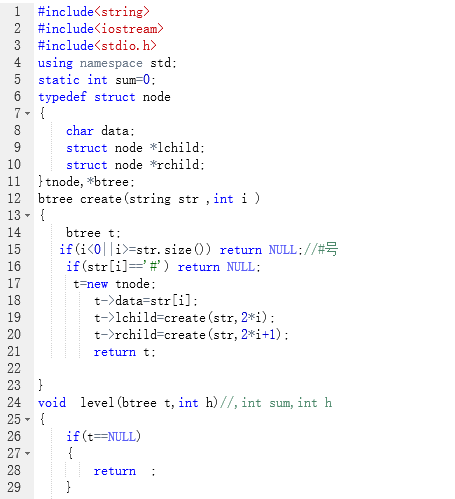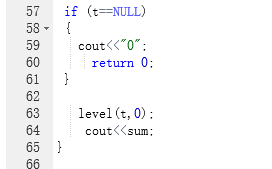### 2.4 PTA提交列表说明。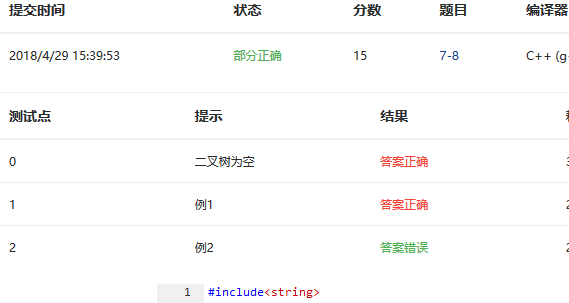• 错误原因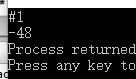• 解决方法
if(i<=0||i>str.size()) return NULL


if(i<=0||i>=str.size()) return NULL


## 3.截图本周题目集的PTA最后排名

### 3.1 PTA排名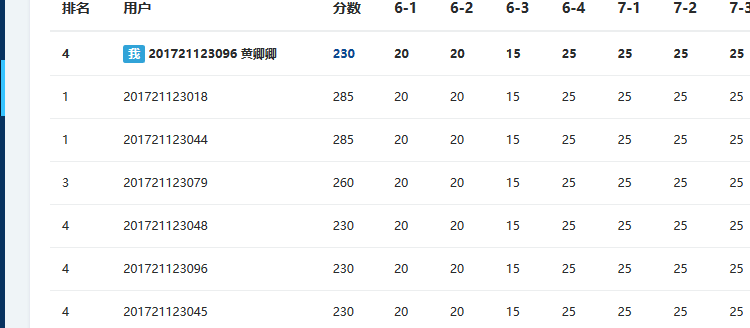## 4. 阅读代码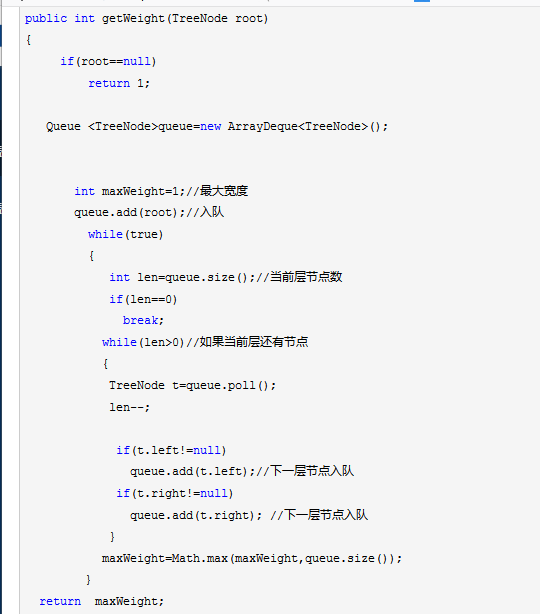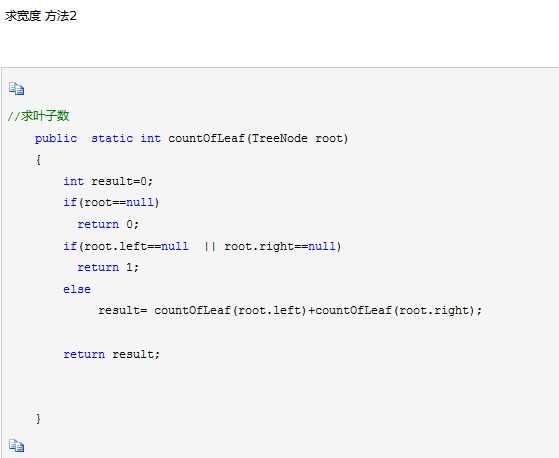## 5. 代码Git提交记录截图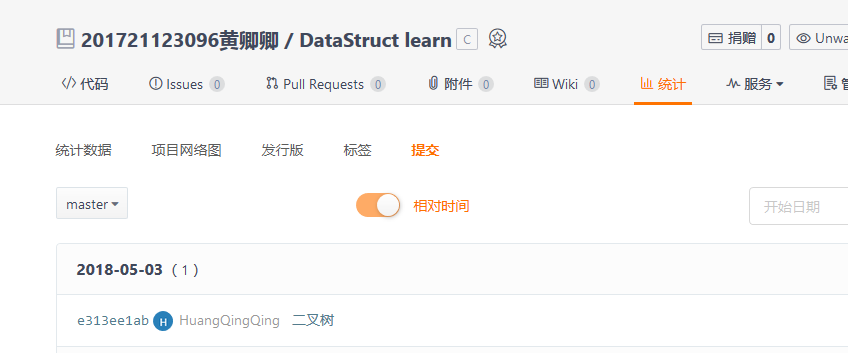posted on 2018-05-05 17:18  开心仔  阅读(197)  评论(0编辑  收藏  举报